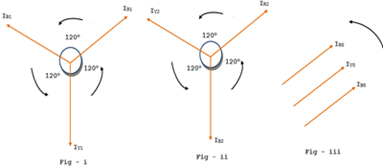# sequence network in power systemThe study of unsymmetrical faults by symmetrical components method can be easily done by drawing sequence network in power system. A sequence network in a particular sequence current in a given power system is the path for the flow of that sequence current in the systems. It is composed of impedances offered to that sequence current in the system, Since there are three sequence currents named Positive, Negative and zero sequence current, there will be three sequence networks in the given power system. They are
1. Positive-sequence network
2. Negative-sequence network
3. Zero-sequence network.

# Positive Sequence Network in Power System

In a given power system the positive sequence network shows all the parts for the flow of positive-sequence currents in the systems. It is represented by one line diagram and is composed if impedances offered to the positive sequence network of a given power systems. The following points may possibly be kept in view:
• Each generator in the system is represented by the generated voltage in series with proper reactance and resistance.
• Current limiting impedances between the generator neutral and ground pass no positive sequence current and so are not included in the positive sequence network.
• All resistances and magnetizing currents for each transformer are neglected as a matter of simplicity.
• For transmission lines, the shunt-capacitances and the resistances are generally neglected
• Motor loads are integrated in the network as generated emf in series with appropriate reactance.

## Negative-Sequence Network in Power System

In a given power system the negative-sequence network shows all the paths for the flow of negative sequence currents in the system. It is also represented by one line diagram and is composed of impedances offered to the negative sequence currents. In the power system the negative-sequence network can be readily obtained from positive sequence network with the following modifications:
• Omit the e.m.fs of 3-phase generators and motors in the positive sequence network. It is because these devices have only positive-sequence generated voltages.
• Change, if necessary, the impedances that represent rotating machines in the positive sequence network. It is because negative sequence impedances of rotating machinery are generally different from that of positive sequence impedances.
• Current limiting impedances between generators neutral and ground pass no negative sequence current and so are not included in the negative sequence network.
• For static devices such as transmission lines and transformers, the negative sequence impedances have the same value as the corresponding positive sequence impedances.

### Zero Sequence Network in Power System

In a given power system the zero sequence networks shows all the paths for the flow of zero-sequence currents. The zero sequence networks depend upon the type of connections of the three phase windings of the components in the system. The following points may be noted about zero sequence networks:
• The zero sequence currents will flow only if there is a return path i.e., path from neutral to ground or to another neutral point in the circuit.
• In the case of a system with no return path for zero sequence currents, these currents cannot exists.

### Reference Bus for Sequence Networks

When we use to draw the sequence networks, it is mandatory to mention the reference potetial w.r.t which all sequences voltage drop are to be considered. For this purpose, we need to keep in mind the below points:
• For positive sequence or the negative sequence networks, the neutral of the generator is considered a s the reference bus. This is logical because positive or negative sequence componens represents the balanced sets and hence all the neutral points must be at the same potential for either any of the positive or negative sequence currents.
• For zero sequence network, the referecne bus is the ground at the generator.2 CommentsComments

#### Read Comment Policy We have Zero Tolerance to Spam. Chessy Comments and Comments with Links will be deleted immediately upon our review.

1.It is composed of impedances offered to that sequence current in the system, Since there are three sequence currents named Positive, Negative and zero sequence current, there will be three sequence networks in the given power system. MB6-895 exam dumps

2.We all love a lot of the talks, My spouse and i truly seasoned, I might like more information in terms of this specific, since it can be wonderful., Using due to find scattering. idm crack